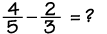Select the correct answer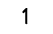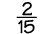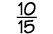Your score Correct — Incorrect —

In order to become skilled in mathematics you need to practice!

Try a workout of 10 problems. If you get at least 8 correct on your first attempt, then you're ready to move on. If not, review "In Depth" and try again.Homework Help | Pre-Algebra | FractionsEmail this page to a friendSearch·  Definitions·  Reducing fractions·  Adding and subtract-     ing fractions·  Multiplying fractions·  Dividing fractions·  Adding and subtract-     ing mixed numbers·  Multiplying     mixed numbers·  Dividing     mixed numbers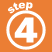First Glance In Depth Examples WorkoutAdding and subtracting fractions